# Kerala Syllabus 9th Standard Physics Solutions Chapter 4 Gravitation

## Kerala State Syllabus 9th Standard Physics Solutions Chapter 4 Gravitation

### Gravitation Textual Questions and Answers

Activity – 1

Lifting a small stone to a certain height and then dropping it downwards.

Kerala Syllabus 9th Standard Physics Notes Chapter 4 Question 1.
What do you observe?
The stone falls down

Hss Live Guru 9th Physics Kerala Syllabus Chapter 4 Question 2.
What could be the reason for the falling of the stone?
Earth’s attraction is the reason

Gravitation Class 9 Kerala Syllabus Chapter 4 Question 3.
What change takes place in the speed of the stone as it is thrown up?
Speed decreases

Gravitation Class 9 State Syllabus Chapter 4 Question 4.
What about the speed when it falls down?
Speed increases

9th Class Physics 4th Lesson Questions And Answers Question 5.
Did you apply any force on the stone to bring it down?
No

9th Class Physics Chapter 4 Kerala Syllabus Question 6.
From where did the stone get the force for the acceleration?
The force required for acceleration got from earth’s attraction

Activity – 2

The stone tied to a thread is suspending from a spring balance.

Hsslive Guru Std 9 Physics Kerala Syllabus Chapter 4 Question 7.
What do you observe?
Spring is stretched downwards

9th Class Physics Notes Kerala Syllabus Chapter 4 Question 8.
The spring stretched down when the stone was suspended from it. Why?
Earth’s attraction is the reason

The earth attracts all objects towards its centre. This force of attraction is the force of gravity.

Conclusion:

• Attraction between two bodies is gravitational force.
• Attraction between bodies and earth is force of gravity.

Kerala Syllabus 9th Standard Physics Notes Pdf Chapter 4 Question 9.
Write down instances where the force of gravity is felt.

• Mango falling naturally form a mango tree.
• Stone which is thrown upwards reaches the ground.
• Raindrops falling downwards.
• When we open the bottle filled with water and keeps it upside down water flows out.
• An object in our hands falls down when it is released.

Activity – 3

Weighing a stone of lower mass and another of higher mass by using a spring balance.
Mass of a body is the amount of matter contained in it

Hsslive Guru 9th Physics Kerala Syllabus Chapter 4 Question 10.
In which case was the reading higher?
Reading of the spring balance is higher when the stone having greater mass is weighed.

Hss Live Guru Class 9 Physics Kerala Syllabus Chapter 4 Question 11.
Which of the stones experienced greater force of attraction of the earth?
Greater force of attraction of the earth is experienced in stone having greater mass.

Physics Chapter 4 Class 9 Notes Kerala Syllabus Question 12.
On the basis of these observations, find out the factor that influences the force of attraction from the earth.
The factor is mass

• As the distance between the bodies increases gravitational force decreases.
• Another factor which influences the gravitational force is distance between the objects.

Universal Law of Gravitation:
All bodies in the universe attract each other. The force of attraction between two bodies is directly proportional to the product of their masses and inversely proportional to the square of the distance between them. If two bodies of masses m1 and m2 are separated by a distance d, thenF ∝ m1, m2 → (1)
F ∝ 1/d2 → (2)
Combining the two, we get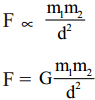G is the gravitational constant.
The value of G is 6.67 x 10-11 Nm2/kg2. The scientist Henry Cavendish determined the value of G for the first time through experiments.

Hss Guru 9 Physics Kerala Syllabus Chapter 4 Question 13.
Complete the table given below, based on Newton’s law of gravitation?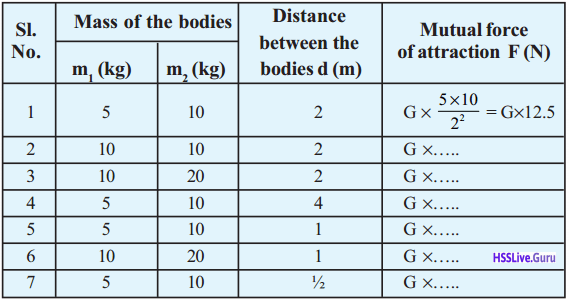Observe the table and find out the answers to the following questions.

Physics Class 9 Chapter 4 Kerala Syllabus Question 14.
Two bodies are at a specific distance so as to attract each other. How many times will the mutual force of attraction be if the mass of one of them is doubled?
Mutual force of attraction doubled

Hss Live 9th Physics Kerala Syllabus Chapter 4 Question 15.
What if the mass of both the bodies are doubled?
Force of attraction becomes 4 times.

Hsslive Guru Class 9 Physics Kerala Syllabus Chapter 4 Question 16.
What if the distance between the bodies is doubled?
When distance is doubled, attractive force reduced as to one fourth (1/4th).

Kerala Syllabus 9th Standard Physics Notes Chapter 4 Question 17.
What happens when the distance between the bodies is halved?
When the distance is halved, force of attraction becomes four times.

9th Physics Notes Kerala Syllabus Chapter 4 Question 18.
Why two children sitting close to each other do not come closer due to mutual force of attraction?
The attractive force between two children sitting close to each other are mutual. The force felt by each of them are equal and very short in magnitude. So they do not come closer to each other.

9th Std Physics Notes Kerala Syllabus Chapter 4 Question 19.
A body of mass 50 kg and another body of mass 60 kg are separated by a distance of 2 m. What is the force of attraction between them?
m1 = 50 kg, m2 = 60 kg
d = 2m, G = 6.67 × 10-11 Nm2/kg2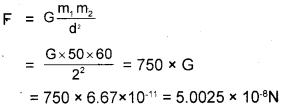Force Of Gravity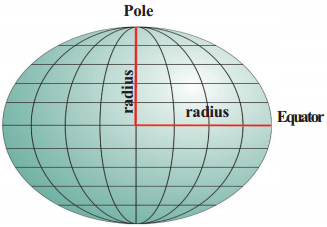Force of attraction by a body on earth GMm
F = $$\frac { GMm }{ R2 }$$
G – Gravitational constant
M – Mass of earth
m – Mass of body

Class 9 Physics Chapter 4 Notes Kerala Syllabus Question 20.
Is the earth really spherical in shape?
No

Question 21.
Is the radius of the earth the same everywhere?
No

Question 22.
Where on the surface of the earth is the radius maximum?
At the equator

Question 23.
Where is it minimum?
At the poles

Question 24.
At which part of the earth must a body be placed so that it will experience the maximum force of attraction? Where the radius is large/where the radius is small?
Where the radius is small. Force of attraction is maximum at the poles.

Question 25.
What change occurs in the force of attraction, if a body is being continuously raised from the surface of the earth?
If a body is being continuously raised from the surface of the earth, force of attraction decreases.

Question 26.
Suppose if the body is moved from the surface of the earth to the centre. What happens?
When it reaches the centre attractive force becomes zero.

Acceleration Due To Gravity

According to II Law of motion.
F = ma
Acceleration due to gravity a = g
i. e, a = g
According to Newton’s law of graviation F = $$\frac { GMm }{ R2 }$$
By Newton’s second law of motion, F = ma = mgQuestion 27.
Find the factors affecting the value of g?

• Mass of the earth

Question 28.
The earth is not a perfect sphere, its radius is not the same everywhere If so, will the value of g be the same everywhere on earth?
Value of g be different everywhere on earth

Question 29.
Where will the value of g be the maximum on the earth’s surface?
At the poles

Question 30.
Where will it be the minimum?
At the equator

Question 31.
What will be the value of g at the centre of the earth?
Zero

Consolidation :

• The value of g on the surface of the earth is 9.8 m/s2,
value of g at pole = 9.78 m/s2
value of g at equator = 9.83 m/s2
• Value of g at moon is 1/6 of the value of g on the earth.

Question 32.
When a stone of mass 50 kg and another of mass 5 kg fall down simultaneously form the top of a five-storey building, which one will reach the ground first?
They reaches the ground at the same time.
Reason: Acceleration due to gravity does not depend on the mass of the body. It will be the same for all
bodies falling to the earth, g = GM/R2

Question 33.
A stone and a sheet of paper are dropped together. Which of the following statements regarding their fall is true?
1. Both of them reach simultaneously
2. The paper reaches first
3. The stone reaches first
The paper reaches slowly
Reason: Resistance of air
Feather and Coin Experiment:
The scientist who discovered that freely falling bodies offers air resistance. This was proved by Issac Newton.
Objects like paper fall slowly Galileo was the first person to; argue that this is due to air resistance. Fie wasn’t able to prove it then because at that time: there were no facilities to create a vacuum. Sir Isaac, Newton could prove this later through the ‘feather and coin’ experiment.

Newton placed a feather and a coin in a long transparent tube with closed ends. The tube was: kept vertical at first and then; suddenly turned upside down.

The coin reached the bottom first followed feather a short while later. The experiment was repeated after removing the air inside the tube and it was found that the feather and the coin reached the bottom simultaneously. The conclusion was that the feather took more time to reach the bottom due to air resistance. Thus Galileo’s argument was proved right.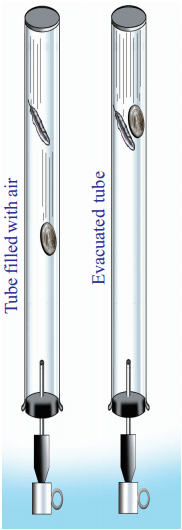Question 34.
When a stone falls, it attract the earth just as the earth attracts the stone. But it is only the stone that falls, the earth does not rise up. Give reason?
Mass of the earth (M) is far greater than that of stone (m). So the acceleration acquired by earth is lesser and that by the stone is greater.

Question 35.
A stone falls down from the top of a wall in 1 s to the ground (g=10 m/s2)
a) What is the speed of the stone just before it touches the ground?
b) Calculate the average speed when the stone is falling down
c) How much is the height of the wall?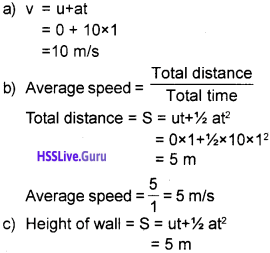Question 36.
A ball thrown vertically upward reached a maximum height of 20m
i) What was the velocity of the stone at the instant of throwing up?
ii) How much time did the ball take to reach the height 20 m?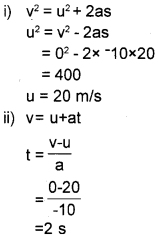Mass and WeightMass is measured using common balance. Spring balance is used to measure weight.

• Mass is measured using common balance.
• The quantity of matter present in an object is called mass.
• Spring balance is used to measure weight.
• Weight is the force of attraction exerted by the earth on an object.
F = mg

Question 37.
Where does a body experience maximum weight on the earth? What is the reason?
Maximum weight experiences at the poles. Because value of g is greater at the poles.

Question 38.
Where on the earth does a body experience minimum weight? What is the reason?
Minium weight experiences at the equator. Value of g is lesser at the equator.

Question 39.
What is the weight of the body when it is at the center of the earth? Give reasons.
At the center, weight is zero. Because value of g is zero

Question 40.
Find out the weight of a body of mass 20 kg. Express the value in newton.
F = mg
= 20 × 9.8
= 196 N

Free Fall

When a body is allowed to fall from a height, it falls to the earth due to the force of gravity. This is free fall.

Question 41.
Suppose a spring balance with a body suspended from it is allowed to fall. What will be the reading shown by the balance?

Question 46.
During the rotation of a giant wheel, a person experiences loss of weight on the descent. Explain why.
Because the giant wheel is in free fall

Question 43.
Why does a freely falling body experience weightlessness? Note it down in your science diary.
Due to free fall, the balance cannot exert a reaction force. Also, the force of gravity is utilized to give acceleration for the body.

Question 44.
What is the weight of a body of mass 10 kg?
Weight F = mg = 10 × 9.8 = 98 N

Question 45.
If this body is allowed to fall freely, will there be any change in the force experienced by the body?
Weight lessens occurs

Question 46.
Drill a hole at the bottom of an open bottle and fill it with water. Water goes out through the hole. Then allow the bottle to fall freely. What do you observe?
During free fall, water and the bottle possess some acceleration. So there is no reaction force experiences in water. As a result water does not go out.

Let Us Assess

Question 1.
If the distance between two bodies that attract each other is trebled, how many times will their mutual force of attraction be? (9 time, 3 times, 1/3, 1/9)
1/9

Question 2.
A body, the mass and the weight of which were already determined at the Equator, is now placed at the Pole. In this context, choose the correct statement from the following:
a) Mass does not change, weight is maximum
b) Mass does not change, weight is minimum
c) Both mass and weight are maximum
d) Both mass and weight are minimum
a) Mass does not change, weight is maximum

Question 3.
Mass of the zeroth is 6 × 1024 kg and that of the Moon is 7.4 × 1022 kg. The distance between Earth and Moon is 3.84 × 105 km. Calculate the force of attraction of Earth of Moon. (G=6.7 × 10-11 Nm2 kg-2)
F = $$\frac { GMm }{ d2 }$$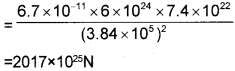Question 4.
a) What is meant by the terms mass and weight?
b) Are they vector of scalar quantities? Why?
c) The mass of a body is 30 kg. What is its weight on earth? (g = 9.8 m/s2)
d) What is its weight on the moon? (g = 1.62 m/s2) )
a) 1. The quantity of matter present in a body is called mass.
2. The force of attraction exerted by earth on a body is referred as weight.
b) Mass is a scalar quantity (No direction)
Weight is a vector quantity (Possess both magnitude and direction)
c) Weight = mg = 30 × 9 = 294 N
d) Weight on the moon = 30 × 1.62 = 48N

Question 5.
If a body of mass 40 kg is kept at a distance of 0.5 m from a body of mass 60 kg, what is the mutual force of attraction between them?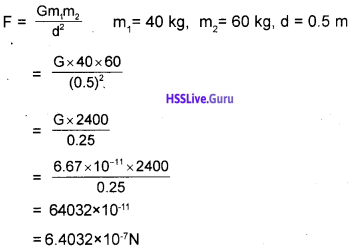Question 6.
Observe the figure and complete the table### Gravitation More Questions and Answers

Question 1.
Complete the tableSI. No. Mass of he bodies Distance between the bodies d(m) Gravitational force F(N) m1kg) m2(kg) 1 12 5 2 G x 15 2 50 20 5 G x 40 3 10 15 0.5 G x 600

Question 2.
How the mass and distance between objects affect gravitational force?
Among the two bodies if the mass of one body is doubled gravitational force becomes two times. When the mass of both the bodies are doubled. Gravitational force becomes four times. When the distance increases gravitational force decreases. Gravitational force varies in reciprocal of the square of the distance between the bodies.

Question 3.
Mass and weight of a body is determined at the pole and at the equator
a) Is there any difference in the mass?
b) Is there any change in the weight?
a) No change in mass
b) Change will occur
c) Mass is the quantity of matter present in a matter. Value of g is greater at the poles and lesser at the equator. So weight varies. Value of g does not affect the mass

Question 4.
Fill in the blanks.
a) Acceleration due to gravity does not affect the ……………. of the object
b) 1 kg wt = ………….. N
a) Mass
b) 9.8

Question 5.
a) What is mean by free fall?
b) Weight of an object in free fall is zero? Why.
c) If a bottle having a hole at the bottom filled with water falling down freely water will not flow out. Why?
a) Falling of a body towards earth due to force of gravity is said to be free fall.
b) In free fall the object cannot be able to give reaction force. Also, the gravitational force is utilized to give acceleration to the object.
c) During free fall, the acceleration of water and bottle remains the same. So no reaction force feel in water.

Question 6.
Correct the following.
a) Force of attraction increases when an object is raised from earth’s surface
b) Value of g remains the same on all regions of earth.
a) Force of attraction decreases when an object is raised from earth’s surface.
b) Value of g is different on all regions of earth.

Question 7.
a) Calculate the weight of a body in the moon if it weights 150 kg in earth?
b) What will be the weight of a body at the center of earth.
a) Weight in the moon = 150 × 1/6 = 25 kg
b) Weight at the center of earth is zero.

Question 8.
a) State Universal Law of gravitation?
b) Represent it mathematically.
c) Mention each letter indicates to what?
d) How mass and distance affect gravitational force?
a) Every bodies in the universe attract each other. This attractive force is directly proportional to the product of their masses and inversely proportional to the square of the distance between them.c) m1 m2 – Mass of the bodies
d – Distance between the objects
F – Gravitational force
G – Gravitational constant
d) Mass increases gravitational force increases Distance increases gravitational force decreases.

Question 9.
List the situations in which gravitational force experiences.